# Test: Sinusoidal Steady State Analysis - Electrical Engineering (EE)

Test Description

## 20 Questions MCQ Test GATE Electrical Engineering (EE) 2024 Mock Test Series - Test: Sinusoidal Steady State Analysis

Test: Sinusoidal Steady State Analysis for Electrical Engineering (EE) 2023 is part of GATE Electrical Engineering (EE) 2024 Mock Test Series preparation. The Test: Sinusoidal Steady State Analysis questions and answers have been prepared according to the Electrical Engineering (EE) exam syllabus.The Test: Sinusoidal Steady State Analysis MCQs are made for Electrical Engineering (EE) 2023 Exam. Find important definitions, questions, notes, meanings, examples, exercises, MCQs and online tests for Test: Sinusoidal Steady State Analysis below.
Solutions of Test: Sinusoidal Steady State Analysis questions in English are available as part of our GATE Electrical Engineering (EE) 2024 Mock Test Series for Electrical Engineering (EE) & Test: Sinusoidal Steady State Analysis solutions in Hindi for GATE Electrical Engineering (EE) 2024 Mock Test Series course. Download more important topics, notes, lectures and mock test series for Electrical Engineering (EE) Exam by signing up for free. Attempt Test: Sinusoidal Steady State Analysis | 20 questions in 60 minutes | Mock test for Electrical Engineering (EE) preparation | Free important questions MCQ to study GATE Electrical Engineering (EE) 2024 Mock Test Series for Electrical Engineering (EE) Exam | Download free PDF with solutions
 1 Crore+ students have signed up on EduRev. Have you?
Test: Sinusoidal Steady State Analysis - Question 1

### In a two element series circuit, the applied voltage and the resulting current are v(t) = 60 + 66sin (10 t) V, i(t) = 2.3sin (103t + 68.3o) A.The nature of the elements would be

Detailed Solution for Test: Sinusoidal Steady State Analysis - Question 1

R-Ccauses a positive phase shift in voltage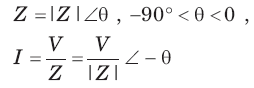Test: Sinusoidal Steady State Analysis - Question 2

### vo (t) = ?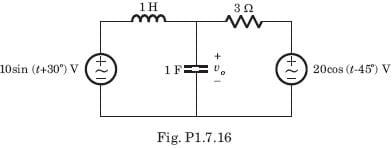Detailed Solution for Test: Sinusoidal Steady State Analysis - Question 2

10 sin (t + 30°) = 10 cos (t - 60°)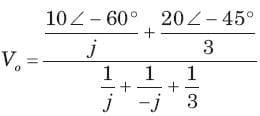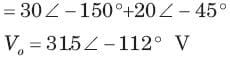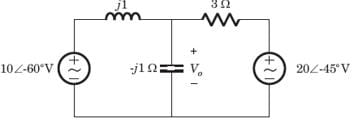Test: Sinusoidal Steady State Analysis - Question 3

### Vo = ?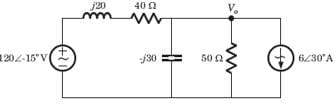Test: Sinusoidal Steady State Analysis - Question 4

The circuit is as shown in fig.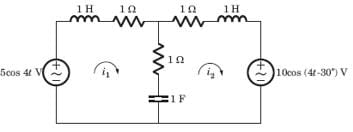i1( t) = ?

Detailed Solution for Test: Sinusoidal Steady State Analysis - Question 4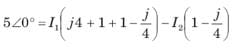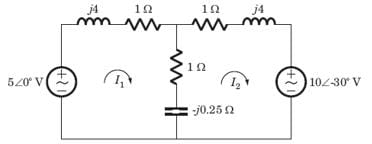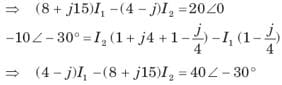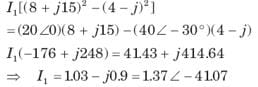Test: Sinusoidal Steady State Analysis - Question 5

The circuit is as shown in fig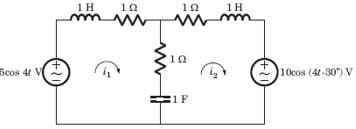i2(t) = ?

Detailed Solution for Test: Sinusoidal Steady State Analysis - Question 5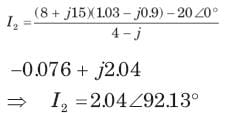Test: Sinusoidal Steady State Analysis - Question 6

Ix = ?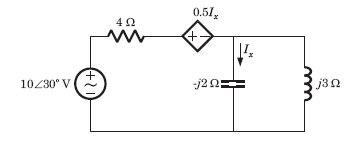Detailed Solution for Test: Sinusoidal Steady State Analysis - Question 6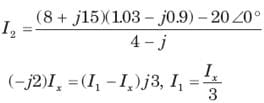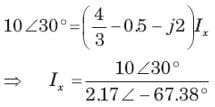Test: Sinusoidal Steady State Analysis - Question 7

Vx = ?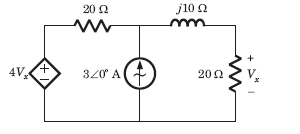Detailed Solution for Test: Sinusoidal Steady State Analysis - Question 7

Let Vo be the voltage across current source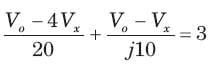Vo(20 + j10) - (20 + j40) Vx = j600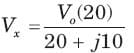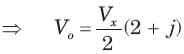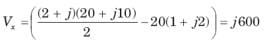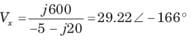Test: Sinusoidal Steady State Analysis - Question 8

Determine the complex power for hte given values in question.

P = 269 W, Q = 150 VAR (capacitive)

Detailed Solution for Test: Sinusoidal Steady State Analysis - Question 8

S = P-jQ = 269-j150 VA

Test: Sinusoidal Steady State Analysis - Question 9

Determine the complex power for hte given valuesin question.

Q = 2000 VAR, pf =09. (leading)

Detailed Solution for Test: Sinusoidal Steady State Analysis - Question 9

pf = cos θ = 0.9 ⇒ θ = 25.84°
Q = S sin θ ⇒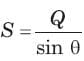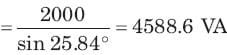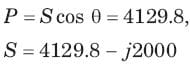Test: Sinusoidal Steady State Analysis - Question 10

Determine the complex power for hte given values in question.

S = 60 VA, Q = 45 VAR (inductive)

Detailed Solution for Test: Sinusoidal Steady State Analysis - Question 10

Q = S sin θ ⇒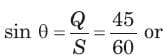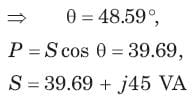Test: Sinusoidal Steady State Analysis - Question 11

Determine the complex power for hte given values in question.

Vrms = 220 V, P = 1 kW, |Z| = 40Ω (inductive)

Detailed Solution for Test: Sinusoidal Steady State Analysis - Question 11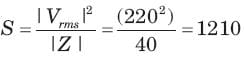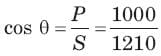= 0.8264 or θ = 34.26°,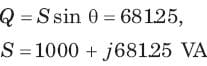Test: Sinusoidal Steady State Analysis - Question 12

Determine the complex power for hte given values in question

Vrms = 21∠20°V, Vrms = 21∠20°V, Irms = 8.5∠-50°A

Detailed Solution for Test: Sinusoidal Steady State Analysis - Question 12

S = Vrms I*rms = (21∠20°)(8.5∠50°)

= 61+j167.7VA

Test: Sinusoidal Steady State Analysis - Question 13

Determine the complex power for hte given values in question.

Vrms = 120∠30°V, Z = 40 + j80Ω

Detailed Solution for Test: Sinusoidal Steady State Analysis - Question 13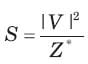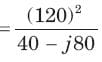= 72 + j144 VA

Test: Sinusoidal Steady State Analysis - Question 14

In a two element series circuit, the applied voltage and the resulting current are v(t) = 60 + 66 sin (1000t) V, i(t) = 2.3sin (1000t + 68.3) 3 A. The nature of the elements would be

Test: Sinusoidal Steady State Analysis - Question 15

A relay coil is connected to a 210 V, 50 Hz supply. If it has resistance of 30Ω and an inductance of 0.5 H, the apparent power is

Detailed Solution for Test: Sinusoidal Steady State Analysis - Question 15

Z= 30 + j(0.5)(2π)(50) = 30 + j157,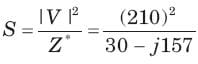Apparent power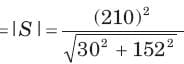= 275.6 VA

Test: Sinusoidal Steady State Analysis - Question 16

In the circuit shown in fig. power factor is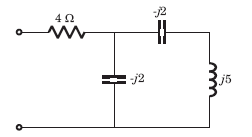Detailed Solution for Test: Sinusoidal Steady State Analysis - Question 16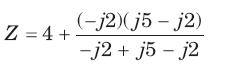= 4 - j6 = 7.21∠ - 56.31°, pf = cos 56.31° = 0.555 leading

Test: Sinusoidal Steady State Analysis - Question 17

The power factor seen by the voltage source is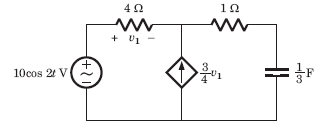Detailed Solution for Test: Sinusoidal Steady State Analysis - Question 17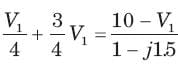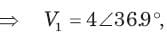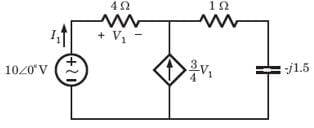I1 = 1∠36.9°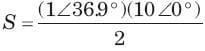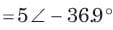pf = cos 36.9° = 0.8 leading

Test: Sinusoidal Steady State Analysis - Question 18

The average power supplied by the dependent source is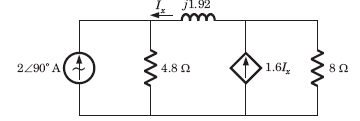Detailed Solution for Test: Sinusoidal Steady State Analysis - Question 18

(2∠ - 90°)4.8 = -Ix (4.8 + j1.92) + 0.6Ix(8)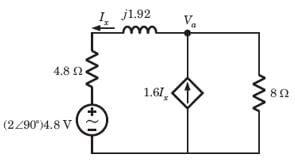Ix = 5∠0°,   Va = 0.6 x 5 x 8 = 24∠0°,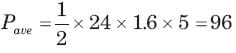Test: Sinusoidal Steady State Analysis - Question 19

In the circuit of fig. the maximum power absorbed by ZL is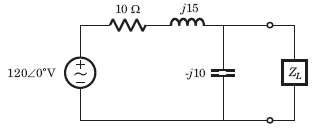Detailed Solution for Test: Sinusoidal Steady State Analysis - Question 19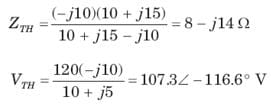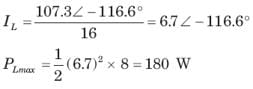Test: Sinusoidal Steady State Analysis - Question 20

The value of the load impedance, that would absorbs the maximum average power is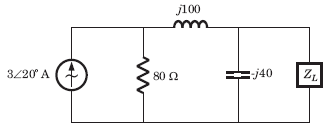Detailed Solution for Test: Sinusoidal Steady State Analysis - Question 20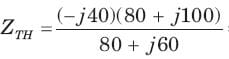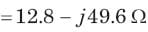## GATE Electrical Engineering (EE) 2024 Mock Test Series

23 docs|285 tests